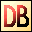DelphiBasics
 CosFunction The Cosine of a number System unit
 function Cos(const Number Extended):Extended;
Description
The Cos function is a mathematical function giving the Cosine value of a Number value given radians.

Notes
The System unit supports only the following angular functions:

ArcTan
Sin
Cos

Related commands
 ArcCos The Arc Cosine of a number, returned in radians ArcSin The Arc Sine of a number, returned in radians ArcTan The Arc Tangent of a number, returned in radians Sin The Sine of a number Tan The Tangent of a numberDownload this web site as a Windows program.

 Example code : Get the Cosine of 60 degrees var   float : single; begin   // The Sine of 60 degrees = 0.5   float := Cos(PI/3);  // = 180/3 = 60 degrees   ShowMessage('Cos(PI/3) = '+FloatToStr(float)); end; Show full unit code Cos(PI/3) = 0.5